Contents >> Applied Mathematics >> Numerical Methods >> Ordinary Differential Equations >> Examples

Ordinary differential equations - Example

Example .

Calculate by the Runge-Kutta’s method integral of the differential equation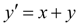at the initial condition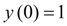on the segment [0, 0.5] with the step of integration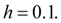S o l u t i o n.  Let’s calculate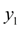. For this purpose at the first we’ll consistently calculate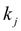: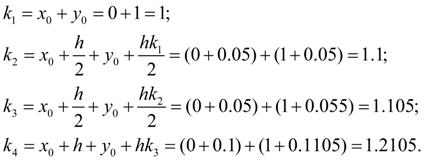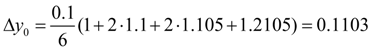and, consequently,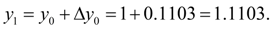The subsequent approaches are similarly calculated. Results of calculations are tabulated:

Results of numerical integration of the differential
equation (1) by the fourth order Runge-Kutta’s method

 i x y k = 0.1 ( x + y ) Δ y 0 1 1 1 0.1 0.05 1.05 1.1 0.22 0.05 1.055 1.105 0.221 0.1 1.1105 1.210 0.1210 1/6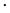0.6620= 0.1103 1 0.1 1.1103 1.210 0.1210 0.15 1.1708 1.321 0.2642 0.15 1.1763 1.326 0.2652 0.2 1.2429 1.443 0.1443 1/60.7947= 0.1324 2 0.2 1.2427 1.443 0.1443 0.25 1.3149 1.565 0.3130 0.25 1.3209 1.571 0.3142 0.3 1.3998 1.700 0.1700 1/60.9415= 0.1569 3 0.3 1.3996 1.700 0.1700 0.35 1.4846 1.835 0.3670 0.35 1.4904 1.840 0.3680 0.4 1.5836 1.984 0.1984 1/61.1034= 0.1840 4 0.4 1.5836 1.984 0.1984 0.45 1.6828 2.133 0.4266 0.45 1.6902 2.140 0.4280 0.5 1.7976 2.298 0.2298 1/61.2828= 0. 2138 5 0.5 1.7974

So , у (0.5) =1.7974.

For comparison the exact decision of the differential equation (1) is: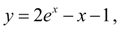whence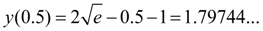Thus, exact and numerical solutions of the equation (1) have coincided up to the fourth decimal place.

The fourth order Runge-Kutta’s method also is widely applied to the numerical solution of systems of ordinary differential equations.

 < Previous Contents Next >